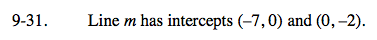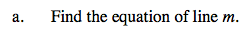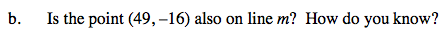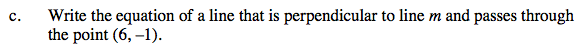### Home > AC > Chapter 9 > Lesson 9.2.1 > Problem9-31

9-31.You are given the y-intercept. Calculate the slope and write the equation.Substitute the given point into the equation you wrote in part (a). Do you get a true statement?The slope of the perpendicular line will be the opposite reciprocal of line m.